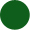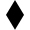# Complexity Explorer Santa Fe Institute

This course is no longer in session.## Nonlinear Dynamics: Mathematical and Computational Approaches

• Introduction to nonlinear dynamics
• Maps and difference equations
• Transients and attractors
• Parameters and bifurcations
• Field trip: Boulder Creek
• Maps I: Unit test
• Return maps
• Constructing the bifurcation diagram
• Exploring the bifurcation diagram
• Feigenbaum and universality
• Field trip: The standard map (with Jim Meiss)
• Maps II: Unit test
• What is a flow?
• State variables and state space
• Introduction to ordinary differential equations (ODEs)
• Nonlinearity and nonintegrability
• Field trip: ODEs and the human insulin system (with Sriram Sankaranarayanan)
• Flows I: Unit test
• Fixed points and stability
• Saddle points and eigenvectors
• Stable and unstable manifolds
• Attractors, strange and otherwise
• Field trip: Using stable and unstable manifolds to design spacecraft trajectories (with Jeff Parker)
• Flows II: Unit test
• ODEs, vector fields, and dynamical landscapes
• Introduction to ODE solvers
• Two simple ODE solvers: forward and backward Euler
• Solving the simple harmonic oscillator ODEs
• Field trip: Systems that can't be modeled with ODEs (with Jean Hertzberg)
• Flows III: Unit test
• ODE solvers, round II: Error and adaptation
• Production ODE solvers
• Numerical dynamics and due diligence
• Shadowing and chaos
• Field trip: Solving partial differential equations (with Christine Hrenya)
• Flows IV: Unit test
• Dynamics and state-space deformation
• Lyapunov exponents
• Sections and projections
• Unstable periodic orbits
• Fractals and chaos
• Field trip: Diffusion-limited aggregation, fractals, and snowflakes (with Dave Feldman)
• Flows V: Unit test
• Time-series analysis and the observer problem
• Delay-coordinate embedding
• Topology, diffeomorphisms, and reconstruction of dynamics
• Estimating embedding parameters
• Caveats and extensions
• Field trip: Detecting extreme events (with Holger Kantz)
• Nonlinear time-series analysis I: Unit test
• Computing fractal dimensions
• Computing Lyapunov exponents
• Noise and filtering
• Field trip: Chaotic mixing and marine invertebrate reproduction (with John Crimaldi)
• Nonlinear time-series analysis II: Unit test
• Prediction
• Control of chaos
• Classical mechanics
• Music and dance (with a coda on the difference between chaos and complexity)
• Applications: Unit test

#### Quiz scores are NOT recorded.

• You may come back to quizzes and take them as many times as you like
• When you are finished, clicking the "Score" button at the bottom of the test will show you the correct responses.

#### Instructions

Homework problems in this course are broken into three types and marked by the following symbols:-- Mandatory problems that should be accessible to all students.-- Optional problems that are somewhat harder.  We strongly suggest that you work through these problems; completing them will give you greater insight into the topics at hand.  Please discuss them and their results on the forum.-- Challenging problems that are intended for experts. These are optional; not all students will be able to complete them. Again, feel free to discuss them on the forum.

In this homework, you'll explore the damped, sinusoidally driven pendulum -- the one in the movie in segment 3.1.  This system is governed by the following system of ODEs:In these equations, Θ is angular position, ω is angular velocity, m is mass,  L is pendulum length, g is acceleration due to gravity, β is the drag coefficient, A is the (drive) amplitude and Ω is the (drive) frequency.  In the driven pendulum in the movie, the amplitude was fixed by the cam follower design and the frequency was controlled by the actuator that drove the motor, but in this problem you'll vary both of those parameters.  For simplicity, we will assume that m = L = 1, which makes the equations look like this:Now point your browswer to:

Pendulum App

To use this application, which simulates the equations above, you need to set the parameter values and initial conditions in the text fields located in the bottom-right panel, then click "Setup". This should be done any time you change the text field values.

To begin the simulation after doing that setup, either click "Start Animation", which will generate a trajectory one step at a time, or click "Generate Full Trajectory", which will generate an n-point-long trajectory, where n is the "Max Trajectory Length" input.

The "Time" panel (bottom left) shows time-series plots of Θ (angular position, shown in yellow) and ω (angular velocity, shown in blue).  The "Phase" panel (top right) shows a state-space portrait, with ω on the y-axis and Θ on the x-axis.